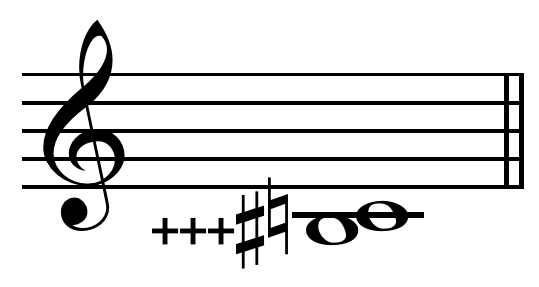#Pythagorean comma

In musical tuning, the Pythagorean comma (or ditonic comma), named after the ancient mathematician and philosopher Pythagoras, is the small interval (or comma) existing in Pythagorean tuning between two enharmonically equivalent notes such as C and B♯ ( Play ), or D♭ and C♯. It is equal to the frequency ratio 531441:524288, or approximately 23.46 cents, roughly a quarter of a semitone (in between 75:74 and 74:73). The comma which musical temperaments often refer to tempering is the Pythagorean comma - wikipediaPythagorean comma on C = B♯+++ (Ben Johnston's notation). 531441:524288 = 23.46 cents. Limit: 3-limit. Note that the note depicted lower on the staff is slightly higher in pitch. - wikimedia- wikimediaPythagorean comma on C = B♯+++ (Ben Johnston's notation). 531441:524288 = 23.46 cents. Limit: 3-limit. Note that the note depicted lower on the staff is slightly higher in pitch. - wikimediaThe Pythagorean comma can be also defined as the difference between a Pythagorean apotome and a Pythagorean limma (i.e., between a chromatic and a diatonic semitone, as determined in Pythagorean tuning), or the difference between twelve just perfect fifths and seven octaves, or the difference between three Pythagorean ditones and one octave (this is the reason why the Pythagorean comma is also called a ditonic comma).

The diminished second, in Pythagorean tuning, is defined as the difference between limma and apotome. It coincides therefore with the opposite of a Pythagorean comma, and can be viewed as a descending Pythagorean comma (e.g. from C♯ to D♭), equal to about −23.46 cents.

In music, harmony is the use of simultaneous pitches (tones, notes), or chords. The study of harmony involves chords and their construction and chord progressions and the principles of connection that govern them. Harmony is often said to refer to the "vertical" aspect of music, as distinguished from melodic line, or the "horizontal" aspect. Counterpoint, which refers to the interweaving of melodic lines, and polyphony, which refers to the relationship of separate independent voices, are thus sometimes distinguished from harmony - wikipedia